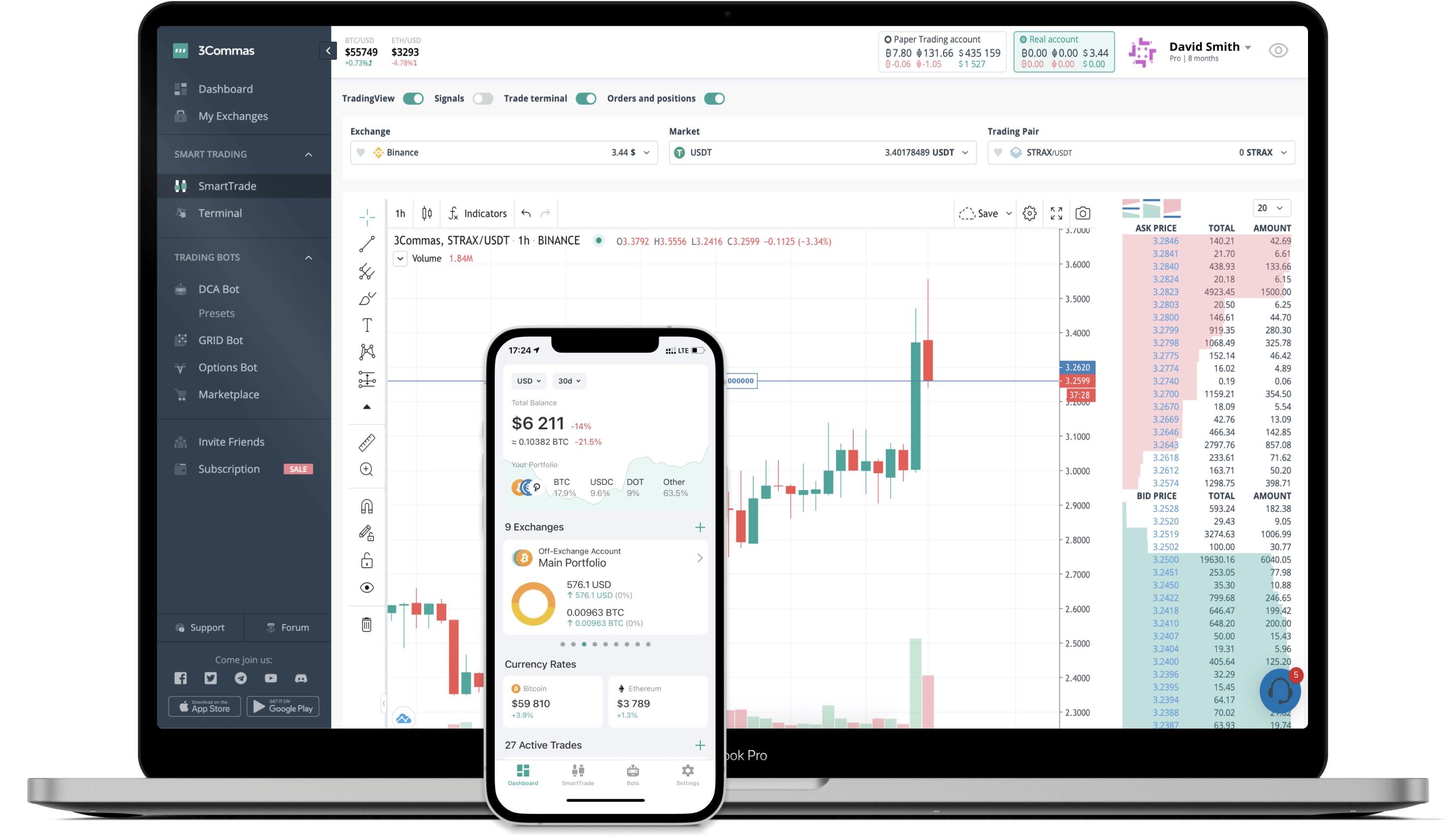ANGLE to BRL Converter

ANGLE
PriceBRL 2.01+1.81%
VolumeBRL 2,370,507
How it works

The 3Commas currency calculator allows you to convert a currency from ANGLE (ANGLE) to Brazilian Real (BRL) in just a few clicks at live exchange rates.

Simply enter the amount of ANGLE you wish to convert to BRL and the conversion amount automatically populates. You can also use our Prices Calculator Table to calculate how much your currency is worth in other denominations, i.e. .1 ANGLE, .5 ANGLE, 1 ANGLE, 5 ANGLE, or even 10 ANGLE.

ANGLE to BRL Exchange Calculator

The chart displays ANGLE price changes in BRL.

The ultimate tools for crypto traders to maximize trading profits while minimizing risk and loss.Latest ANGLE to BRL price calculator

ANGLE market price is updated every three minutes and is automatically displayed in BRL. Below are the most popular denominations to convert to BRL.

ANGLE
Brazilian Real
0.01
ANGLE
0.02
BRL
0.1
ANGLE
0.20
BRL
1
ANGLE
2.01
BRL
2
ANGLE
4.02
BRL
3
ANGLE
6.03
BRL
5
ANGLE
10.05
BRL
10
ANGLE
20.10
BRL
25
ANGLE
50.25
BRL
50
ANGLE
100.50
BRL
100
ANGLE
201.00
BRL
250
ANGLE
502.50
BRL
500
ANGLE
1005.00
BRL
1000
ANGLE
2010.00
BRL
2500
ANGLE
5025.00
BRL
Brazilian Real
ANGLE
0.01
BRL
0.00497512
ANGLE
0.1
BRL
0.04975124
ANGLE
1
BRL
0.49751244
ANGLE
2
BRL
0.99502488
ANGLE
3
BRL
1.49253731
ANGLE
5
BRL
2.48756219
ANGLE
10
BRL
4.97512438
ANGLE
25
BRL
12.43781095
ANGLE
50
BRL
24.87562189
ANGLE
100
BRL
49.75124378
ANGLE
250
BRL
124.37810945
ANGLE
500
BRL
248.75621891
ANGLE
1000
BRL
497.51243781
ANGLE
2500
BRL
1243.78109453
ANGLE

Top Currencies

The price of ANGLE in other fiat and cryptocurrencies is based on the corresponding exchange rate of those currencies to the Brazilian Real.

FAQ

How much is 1 ANGLE in Brazilian Real?

ANGLE price in BRL is constantly changing.

At this moment, 1 ANGLE equals 2.01 BRL

How do I calculate the conversion from ANGLE to BRL?

The 3Commas ANGLE Calculator allows you to easily calculate the conversion price of ANGLE to BRL by simply entering the amount of ANGLE in the corresponding field and will automatically convert the value in Brazilian Real (BRL).

You can also use our ANGLE price table above to check the latest ANGLE price in major fiat and crypto currencies.

How can I convert ANGLE to BRL?

The most common way of converting ANGLE to BRL is by using a Crypto Exchange or a P2P (person-to-person) exchange platform like LocalBitcoins, etc.